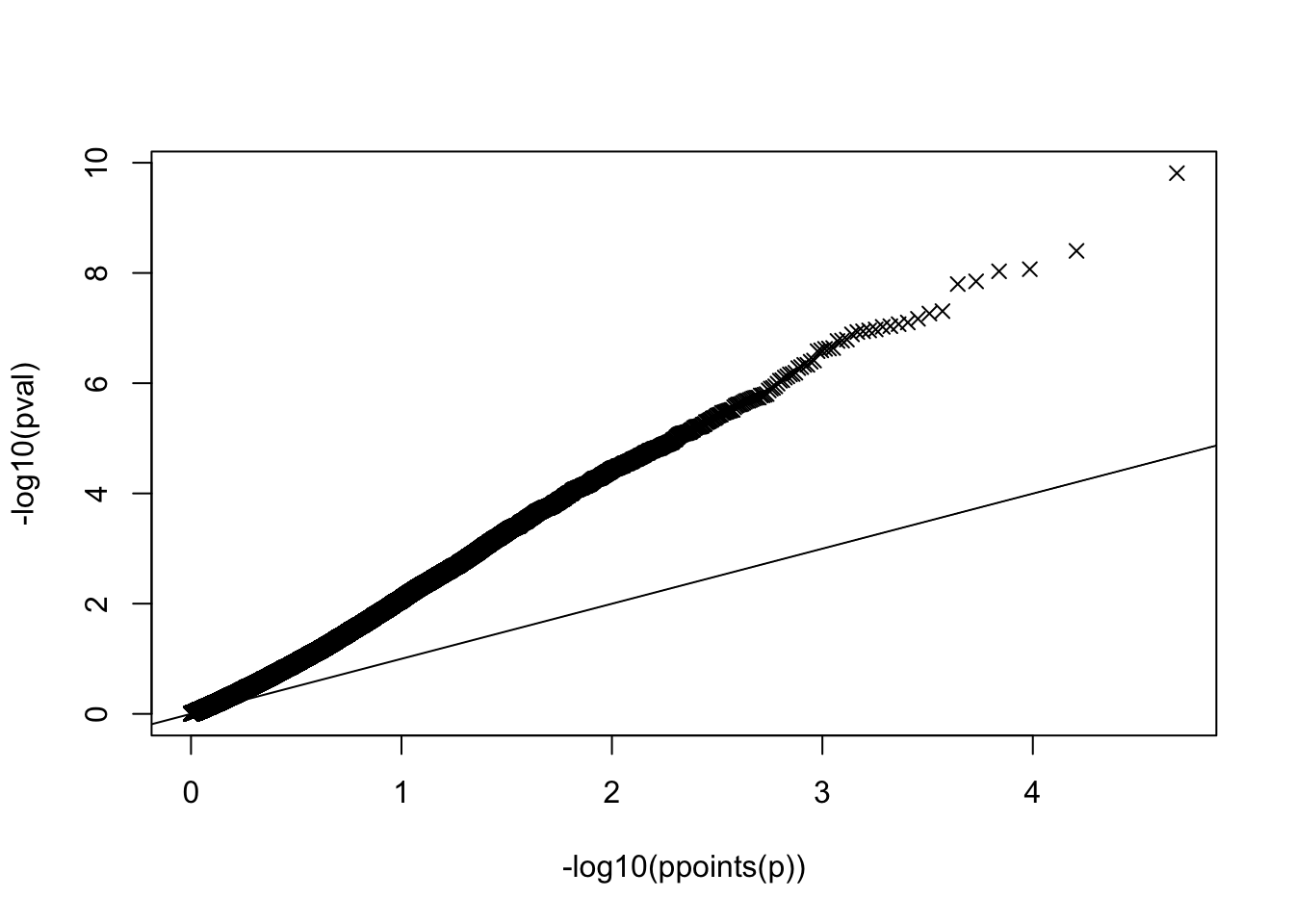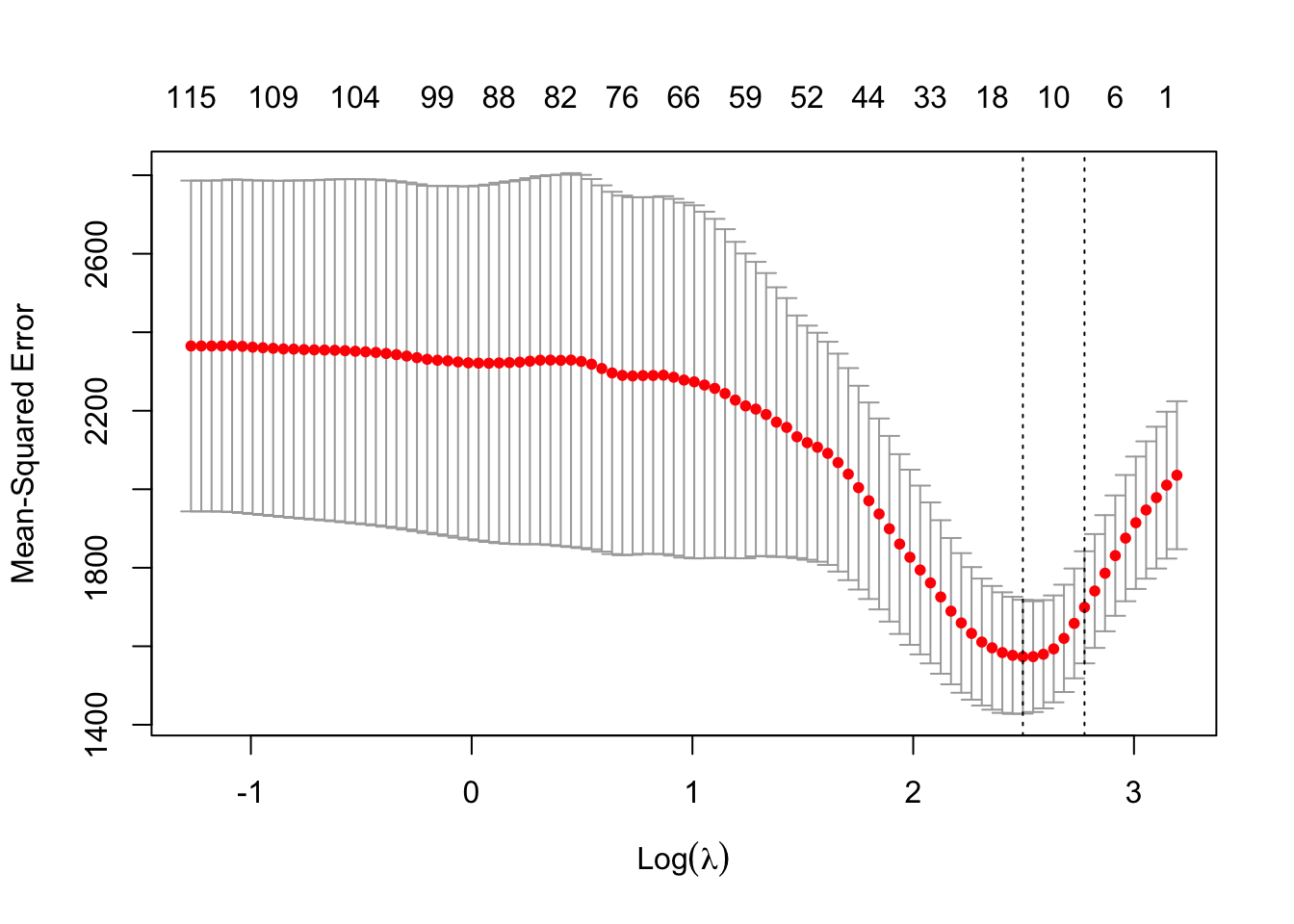Let’s look at a study of breast cancer metastasis published by Van’t Veer et al. in 2002. Data (15 MB) were downloaded from http://web.as.uky.edu/statistics/users/pbreheny/603/ and can now be found from https://www.mv.helsinki.fi/home/mjxpirin/HDS_course/material/vantveer.txt.

In the study, biological samples were obtained from the tumors of women with breast cancer. These samples were scanned with a microarray, that measures the expression of 10000s of genes simultaneously (i.e how much of the gene product is being produced by the cells in the sample). The patients were followed up to see how long it took for the cancer to metastasize (spread elsewhere, which is bad). Clinically, the goal is to identify patients with poor prognosis in order to administer more aggressive follow-up treatment for them. Scientifically, knowledge of the genes related to worse outcome can help understand the disease better, and to develop some therapeutics in the future.

set.seed(21)
dim(D) #rows patients, cols outcome + gene expressions
##     98 24189
anyNA(D)
##  FALSE
y = as.numeric(D[,1]) #survival time
X = as.matrix(D[,2:ncol(D)]) #gene expression measurements
rm(D)
n = length(y)
p = ncol(X)
summary(y)
##    Min. 1st Qu.  Median    Mean 3rd Qu.    Max.
##    1.00   25.25   56.50   63.83   97.00  161.00
summary(apply(X, 2, mean)) #not mean centered
##      Min.   1st Qu.    Median      Mean   3rd Qu.      Max.
## -1.409847 -0.023194  0.006776 -0.003500  0.032255  0.313347
summary(apply(X, 2, sd)) #not standardized
##    Min. 1st Qu.  Median    Mean 3rd Qu.    Max.
## 0.03919 0.11772 0.16494 0.19807 0.24711 1.08625

A filtering step could be to remove predictors that have small variance, because these are less likely to be informative in statistical sense. Of course, any gene could be important biologically even if it varies only a little between individuals, but we have less statistical power to find such effects, and therefore, if we needed to filter out predictors, these could be candidates. Our methods are efficient enough so, for now, let’s just keep all in and standardize the columns.

X = scale(X) #now mean=0 var=1 for each column
y = y - mean(y) #mean center, but do not scale to keep the time interpretation.
#we could now ignore the intercept because everything is mean centered

Let’s first do a quick version of ordinary least squares univariately for each gene $$j$$ using the model $$y = x_j \beta_j + \varepsilon$$. Now we do not want to do a for-loop to fit lm() for 24000+ times but we use formulae from Exercise 1.5.

After standardization within the sample $$\pmb x_j^T \pmb x_j=n-1$$ for each column $$j$$ and so $\widehat{\beta}_j =\frac{\pmb x_j^T \pmb y}{\pmb x_j^T \pmb x_j} = \frac{\pmb x_j^T \pmb y}{n-1}.$ Thus the vector $$\widehat{\pmb \beta}^{(UNI)} = \pmb X^T \pmb y/(n-1)$$ has the univariate least squares estimates, and this can be computed by a single matrix-by-vector operation, so is as quick as it can get.

Similarly, we can compute the univariate estimate of $$\sigma^2_j$$ for each gene as $\widehat{\sigma^2}_j = \frac{1}{n-2} \left (\pmb y- \pmb x_j \widehat{\beta}_j\right)^T \left(\pmb y- \pmb x_j \widehat{\beta}_j\right)$

and then we have the standard erros as $s_j = \sqrt{\frac{\widehat{\sigma^2}_j}{(n-1)}}.$

beta.uni = as.vector((t(X)%*%y)/(n-1))
sigma2.uni = colSums(( y - t( t(X)*beta.uni) )^2)/(n-2) #this is sigma2 formula above written in R
se = sqrt(sigma2.uni/(n-1))
#Now we have fit 24000+ linear models.
#Check an arbitrary column against lm() output:
i = 10625
summary(lm(y ~ X[,i]))$coefficients[2,1:2] ## Estimate Std. Error ## -5.682152 4.539231 c(beta.uni[i], se[i]) ## Gene10625 ## -5.682152 4.539231 #OK. pval = pchisq( (beta.uni/se)^2, df = 1, lower = F) qqplot(-log10(ppoints(p)), -log10(pval), pch = 4) #huge deviations abline(0,1)summary(pval) ## Min. 1st Qu. Median Mean 3rd Qu. Max. ## 0.00000 0.07208 0.29467 0.36428 0.62452 0.99990 Let’s fit LASSO model to the data. library(glmnet) ## Loading required package: Matrix ## Loaded glmnet 4.1-3 cv.lasso = cv.glmnet(X, y, alpha = 1) #takes < 5 seconds even with p>20000 plot(cv.lasso)plot(cv.lasso$glmnet.fit, xvar = "lambda")
abline( v = log(cv.lasso$lambda.min), lty = 2 ) abline( v = log(cv.lasso$lambda.1se), lty = 2 )